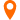首页W3C技术头条python正则表达式re.search()怎么使用？

# python正则表达式re.search()怎么使用？

w3cschool小编 2021-07-22 11:43:42 浏览数 (64)

### 1 re.search() 的作用:

re.search会匹配整个字符串，并返回第一个成功的匹配。如果匹配失败，则返回None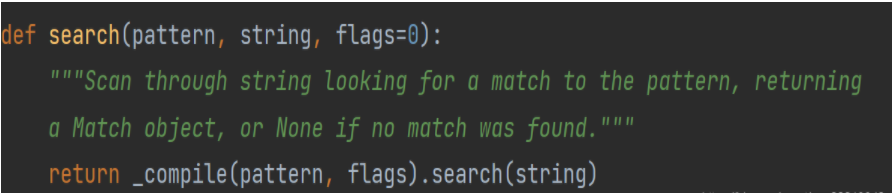pattern: 匹配的规则,

string : 要匹配的内容,

flags 标志位 这个是可选的,就是可以不写,可以写, 比如要忽略字符的大小写就可以使用标志位

flags 的主要内容如下

flags : 可选，表示匹配模式，比如忽略大小写，多行模式等，具体参数为：

1. re.I 忽略大小写
2. re.L 表示特殊字符集 w, W, , B, s, S 依赖于当前环境
3. re.M 多行模式
4. re.S 即为 . 并且包括换行符在内的任意字符（. 不包括换行符）
5. re.U 表示特殊字符集 w, W, , B, d, D, s, S 依赖于 Unicode 字符属性数据库
6. re.X 为了增加可读性，忽略空格和 # 后面的注释

### 2 demo 练习re.search() 的使用

2.1  search 简单的匹配

``````import re

content = "abcabcabc"
rex = re.search("c", content)
print(rex)``````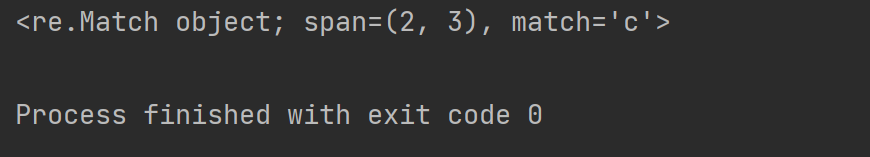match 是匹配的内容, 内容为c

2.2 获取匹配的下标

``````import re

content = "abcabcabc"
rex = re.search("c", content)
print(rex.group())``````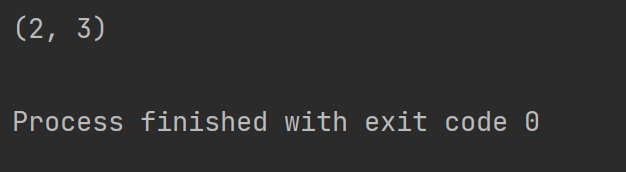2.3 获取匹配的内容 ,使用group(匹配的整个表达式的字符串)

``````import re

content = "abcabcabc"
rex = re.search("c", content)
print(rex.group())``````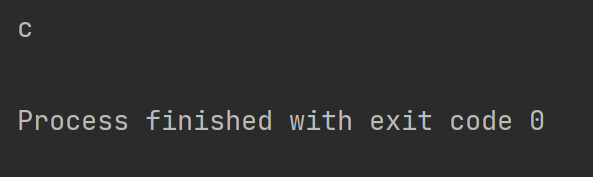2.4 使用标志位忽略匹配的大小写

``````import re

content = "abcabcabc"
rex = re.search("C", content, re.I)
print(rex.group())``````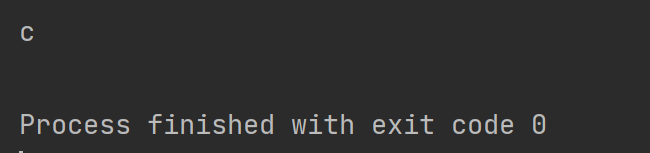2.5 使用search 匹配字符串里面的数组

``````import re

content = "abc123abc"
rex = re.search("d+", content)
print(rex.group())``````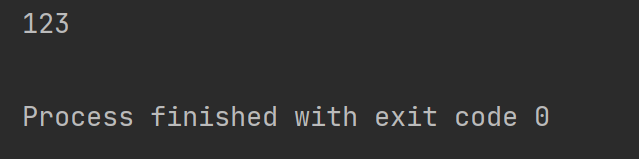2.6 search 结合compile 使用

``````import re

content = "abc123abc"
rex_content = re.compile("d+")
rex = rex_content.search(content)
print(rex.group())``````2.7 group 的使用

``````import re

content = "abc123def"
rex_compile = re.compile("([a-z]*)([0-9]*)([a-z]*)")
rex = rex_compile.search(content)
print(rex.group())
print(rex.group(0))  # group()和group(0) 一样匹配的是整体
print(rex.group(1))  # 匹配第一个小括号的内容
print(rex.group(2))  # 匹配第二个小括号的内容
print(rex.group(3))  # 匹配第三个小括号的内容``````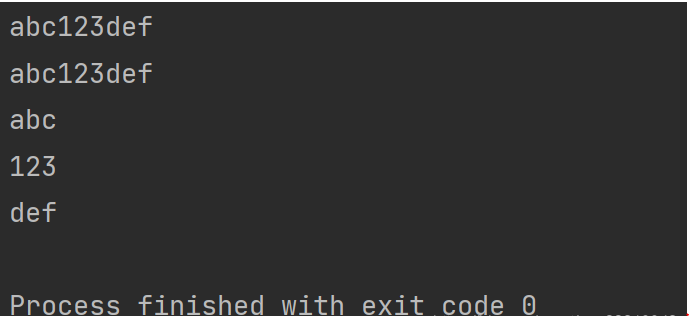group() 小括号里面不止有数字,可以是自定的内容如下

``````content = "zhangsanfeng108le"
rex_compile = re.compile("(?P<name>[a-z]*)(?P<age>[0-9]*)")
rex_content = rex_compile.search(content)
print(rex_content.group())
print(rex_content.group("name"))  # 这里效果等同于group(1)
print(rex_content.group("age"))  # 这里效果等同于group(2)``````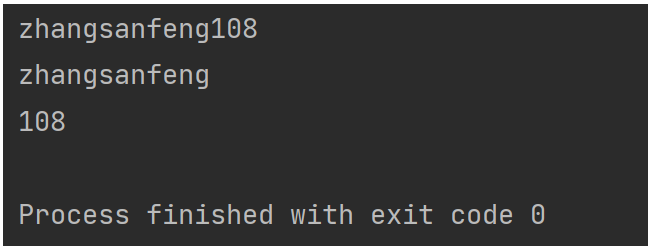### {{ relateCourse.bookname }}

{{ relateCourse.description }}

{{ relateCourse.watch_count }} 人参与